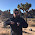Monday, December 10, 2018

JOLTS day

Checking in on the dynamic information equilibrium model forecasts, and everything is pretty much status quo. Job openings are still on a biased deviation. Click to enlarge.

Based on this model which puts hires as a leading indicator, we should continue to see the unemployment rate fall through March of 2019 (5 months from October 2018):

The dashed line shows a possible recession counterfactual (with average magnitude and width, i.e. steepness) constrained by the fact that the JOLTS hires data is not showing any sign of a shock.

Here's the previous update from November (with September 2018 JOLTS data).

1.Hi, I was trying to beat you to the punch and update the data myself. So I used this notebook and went through it, fixing the various things... for some reason the "SinglePredictionBands" thing blows up and produces a formula with coefficients of 10^9689 and similar in it. I'm not sure if you've been running into that problem and fixed it or if it's just that something changed from Mathematica 10.3 to 11.3.

Anyways, I got a bit sidetracked and spent some time analyzing your entropy-minimization code. I noticed it's very sensitive to the size of the bins used. And I'm not sure what kind of entropy it's actually minimizing; the paper and the Python code say relative entropy, but it doesn't match the Wikipedia page on relative entropy; the standard form is $\sum _{i}P(i)\log \left({\frac {P(i)}{Q(i)}}\right)$ whereas yours is something like $\sum _{i}P(i)\left({\frac {\log P(i)}{\log Q(i)}}\right)$ (cross fingers the MathJax works...). Am I missing something?

1.I added the mathjax to this post so your equations work. I'm glad someone else is going through the codes and checking! Thank you.

So I've always referred to that standard measure as the divergence or KL divergence, so to me "relative entropy" is not a well-defined concept. Apparently wikipedia redirects to KL divergence, but then wikipedia gets the description of information as an expectation value wrong. Anyway, I took the liberty of appropriating it since that seemed like a good description. What I actually compute is the ratio of the entropy of the histogram bin to the entropy of a uniform distribution (which is the maximum entropy distribution with the given constraints, so represents a theoretical maximum for the histogram distribution resulting in values that are less than one). I'm interesting in the difference between this and the KL divergence. Working through the math, it seems

$\exp D_{KL} = \frac{\exp H(p_{i})}{\exp H(q)}$

for a uniform distrubtion $q$, so the measure I used effectively took the log of the numerator and denominator of the RHS. Since these are both positive (i.e. information entropy), the ratio (exp A)/(exp B) is going to have the same behavior as A/B (what I used), but with a larger variance.

It's true that it's very sensitive to the bin size, and I usually adjust it to both a) to be about the size of the noise in the data (so that most fluctuations around a straight line fall in a single bin) and b) to get a nice roughly quadratic local minimum. As with most numerical methods, it can be part art, part science. But if I fail to get approximately the same minimum for a few different bin sizes, then I'll resort to other methods such as fitting the slope along with the the shocks as one big optimization. That method has the problem of coupling the dynamic equilibrium slope and the shock parameters, which can be problematic in other ways.

And yes, I don't know why single prediction bands produces such weird coefficients, but I've tested them numerically using simulated data and they seem correct. I've used that function for far more important things in my real job, so I made sure to test it out to be certain it wasn't giving me garbage.